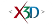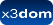## Node: GeoElevationGrid

X3D: 3.3
Component: Geospatial
Status:
experimental

➨ Fields
The GeoElevationGrid node specifies a uniform grid of elevation values within some spatial reference frame. These are then transparently transformed into a geocentric, curved-earth representation.

### HTML Encoding and Default Values⤴

``` <GeoElevationGrid ccw='true' creaseAngle='0' geoGridOrigin='0,0,0' geoOrigin='x3dom.nodeTypes.GeoOrigin' geoSystem='['GD','WE']' height='0,0' lit='true' metadata='X3DMetadataObject' solid='true' texCoord='x3dom.nodeTypes.X3DTextureCoordinateNode' useGeoCache='true' xDimension='0' xSpacing='1.0' yScale='1' zDimension='0' zSpacing='1.0' ></GeoElevationGrid> ```

### Fields⤴

These are the X3D / X3DOM fields of this node. Values should usually be received / set as strings via DOM functions (i.e., using `setAttribute("myFieldName", "myFieldValue")` and `getAttribute("myFieldName")`).
Name Type Default Value Range Inheritance Standard Description
ccw SFBool trueThe ccw field defines the ordering of the vertex coordinates of the geometry with respect to user-given or automatically generated normal vectors used in the lighting model equations.
creaseAngle SFDouble 0 [0, inf]The creaseAngle field affects how default normals are generated. If the angle between the geometric normals of two adjacent faces is less than the crease angle, normals shall be calculated so that the faces are shaded smoothly across the edge; otherwise, normals shall be calculated so that a lighting discontinuity across the edge is produced. Crease angles shall be greater than or equal to 0.0 angle base units.
geoGridOrigin SFVec3d 0,0,0The geoGridOrigin field specifies the geographic coordinate for the south-west corner (bottom-left) of the dataset.
geoOrigin SFNode GeoOriginThe geoOrigin field is used to specify a local coordinate frame for extended precision.
geoSystem MFString ['GD','WE'] {["GD", ...], ["UTM", ...], ["GC", ...]}The geoSystem field is used to define the spatial reference frame.
height MFDouble 0,0The height array contains xDimension × zDimension floating point values that represent elevation above the ellipsoid or the geoid, as appropriate. These values are given in row-major order from west to east, south to north.
lit SFBool trueSpecifies whether this geometry should be rendered with or without lighting.
metadata SFNode X3DMetadataObject Core/X3DNodeField to add metadata information
solid SFBool true Rendering/X3DGeometryNodeSpecifies whether backface-culling is used. If solid is TRUE only front-faces are drawn.
texCoord SFNode Texturing/X3DTextureCoordinateNodeThe texCoord field specifies per-vertex texture coordinates for the GeoElevationGrid node. If texCoord is NULL, default texture coordinates are applied to the geometry.
useGeoCache SFBool true Rendering/X3DGeometryNodeMost geo primitives use geo cache and others might later on, but one should be able to disable cache per geometry node. Defaults to global useGeoCache setting parameter.
xDimension SFInt32 0 [0, inf]Defines the grid size in x.
xSpacing SFDouble 0 [0, inf]When the geoSystem is "GD", xSpacing refers to the number of units of longitude in angle base units between adjacent height values. When the geoSystem is "UTM", xSpacing refers to the number of eastings (length base units) between adjacent height values
yScale SFFloat 1 [0, inf]The yScale value can be used to produce a vertical exaggeration of the data when it is displayed. If this value is set greater than 1.0, all heights will appear larger than actual.
zDimension SFInt32 0 [0, inf]Defines the grid size in z.
zSpacing SFDouble 0 [0, inf]When the geoSystem is "GD", zSpacing refers to the number of units of latitude in angle base units between vertical height values. When the geoSystem is "UTM", zSpacing refers to the number of northings (length base units) between vertical height values.